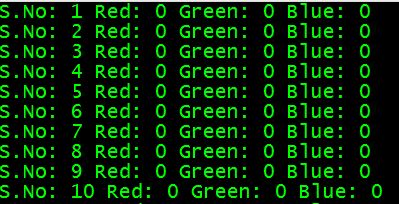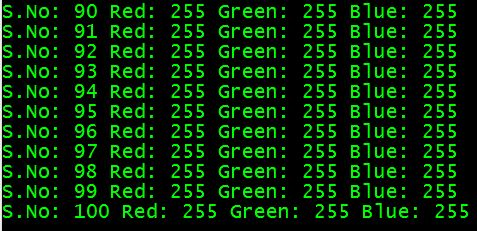# Java DIP - Image Pixels

An image contains a two dimensional array of pixels. It is actually the value of those pixels that make up an image. Usually an image could be color or grayscale.

In Java, the BufferedImage class is used to handle images. You need to call getRGB() method of the BufferedImage class to get the value of the pixel.

## Getting Pixel Value

The pixel value can be received using the following syntax−

```Color c = new Color(image.getRGB(j, i));
```

## Getting RGB Values

The method getRGB() takes the row and column index as a parameter and returns the appropriate pixel. In case of color image, it returns three values which are (Red, Green, Blue). They can be get as follows−

```c.getRed();
c.getGreen();
c.getBlue();
```

## Getting Width and Height of Image

The height and width of the image can be get by calling the getWidth() and getHeight() methods of the BufferedImage class. Its syntax is given below−

```int width = image.getWidth();
int height = image.getHeight();
```

Apart from these methods, there are other methods supported in the BufferedImage class. They are described briefly−

Sr.No. Method & Description
1

copyData(WritableRaster outRaster)

It computes an arbitrary rectangular region of the BufferedImage and copies it into a specified WritableRaster.

2

getColorModel()

It returns ColorModel of an image.

3

getData()

It returns the image as one large tile.

4

getData(Rectangle rect)

It computes and returns an arbitrary region of the BufferedImage.

5

getGraphics()

This method returns a Graphics2D, but is here for backwards compatibility.

6

getHeight()

It returns the height of the BufferedImage.

7

getMinX()

It returns the minimum x coordinate of this BufferedImage.

8

getMinY()

It returns the minimum y coordinate of this BufferedImage.

9

getRGB(int x, int y)

It returns an integer pixel in the default RGB color model (TYPE_INT_ARGB) and default sRGB colorspace.

10

getType()

It returns the image type.

### Example

The following example demonstrates the use of java BufferedImage class that displays pixels of an image of size (100 x 100)−

```import java.awt.*;
import java.awt.image.BufferedImage;

import java.io.*;

import javax.imageio.ImageIO;
import javax.swing.JFrame;

class Pixel {
BufferedImage image;
int width;
int height;

public Pixel() {
try {
File input = new File("blackandwhite.jpg");
width = image.getWidth();
height = image.getHeight();

int count = 0;

for(int i=0; i<height; i++) {

for(int j=0; j<width; j++) {

count++;
Color c = new Color(image.getRGB(j, i));
System.out.println("S.No: " + count + " Red: " + c.getRed() +"  Green: " + c.getGreen() + " Blue: " + c.getBlue());
}
}

} catch (Exception e) {}
}

static public void main(String args[]) throws Exception {
Pixel obj = new Pixel();
}
}
```

### Output

When you execute the above example, it would print the pixels of the following image −

Original ImagePixels OutputIf you scroll down the ouput, the following pattern is seen−